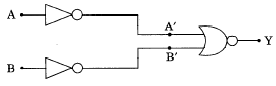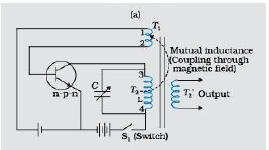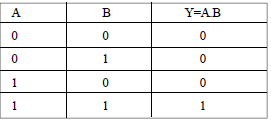(a) Explain briefly the principle on which a transistor-amplifier works as an oscillator, Draw the necessary circuit diagram and explain its working.

(b) Identify the equivalent gate for the following circuit and write its truth table.a) Principle : A portion of the output power is returned back (feedback) to the input in phase with the starting power (This process is termed as positive feedback)                             (1/2) marks(1 $1}{2}$)

Working:

As the switch ${\mathrm{S}}_{1}$ is put on, a surge of collector current flows in the transistor. This current flows through the coil ${\mathrm{T}}_{2}$. The inductive coupling between coil ${\mathrm{T}}_{2}$ and coil ${\mathrm{T}}_{1}$ now causes a current to flow in the emitter circuit . As a result of this positive feedback, the emitter current also increases . When the current in ${\mathrm{T}}_{2}$ becomes saturated , it stops increasing. Now emitter current begins to fall and becomes minimum. The whole process keeps on repeating itself.                           (1) marks

b) AND Gate(1) marks

Difficulty Level: